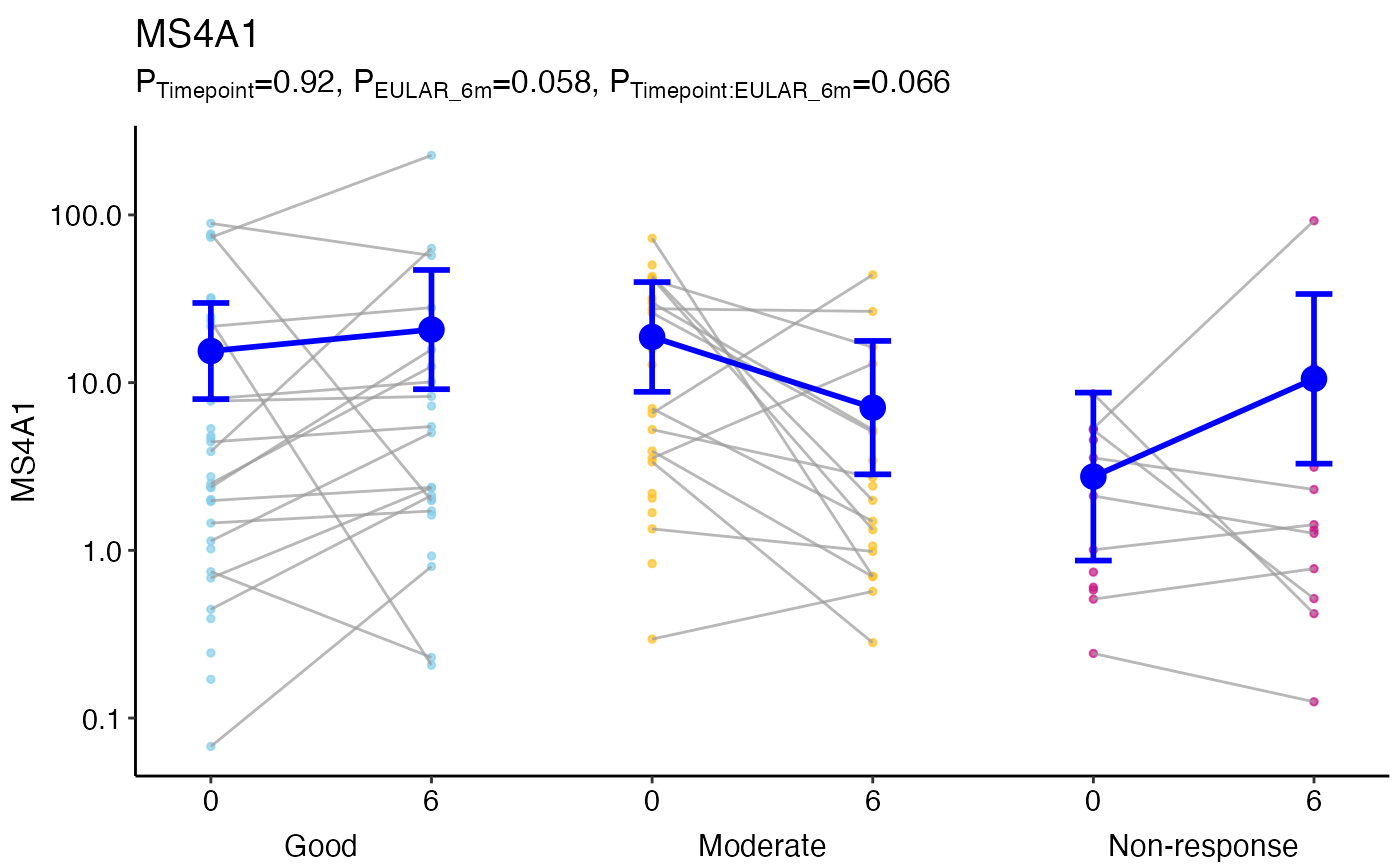Plot to show differences between groups and over time using ggplot2.

## Usage

ggmodelPlot(
object,
geneName = NULL,
x1var = NULL,
x2var = NULL,
x2shift = NULL,
xlab = NULL,
ylab = geneName,
plab = NULL,
title = geneName,
logTransform = is(object, "GlmmSeq"),
shapes = 19,
colours = "grey60",
lineColours = "grey60",
markerSize = 1,
fontSize = 12,
alpha = 0.7,
x2Offset = 5,
modelSize = 4,
modelColours = "blue",
modelLineSize = 1,
modelLineColours = modelColours,
...
)

## Arguments

object

A glmmSeq/lmmSeq object created by glmmSeq::glmmSeq() or glmmSeq::lmmSeq()

geneName

The gene/row name to be plotted

x1var

The name of the first (inner) x parameter, typically 'time'. This is anticipated to have different values when matched by ID.

x2var

The name of an optional second (outer) x parameter, which should be a factor.

x2shift

Amount to shift along x axis for each level of x2var. By default the function will arrange each level of x2var side by side.

xlab

Title for the x axis

ylab

Title for the y axis

plab

Optional character vector of labels for p-values. These must align with column names in object@stats\$pvals.

title

Plot title. If NULL gene name is used

logTransform

Whether to perform a log10 transform on the y axis

shapes

The marker shapes (default=19)

colours

The marker colours as vector

lineColours

The line colours (default='grey60') as vector

markerSize

Size of markers (default=1)

fontSize

Plot font size

alpha

Line and marker opacity (default=0.7)

x2Offset

Vertical adjustment to secondary x-axis labels (default=5)

Whether to add underlying data points (default=TRUE)

Whether to add the fit model with markers (default=TRUE)

modelSize

Size of model points (default=4)

modelColours

Colour of model fit markers (default="blue") as vector

modelLineSize

Size of model points (default=1) as vector

modelLineColours

Colour of model fit lines

Logical whether to add boxplots for mean and IQR

...

Other parameters to pass to ggplot2::theme().

## Value

Returns a paired plot for matched samples.

## Examples

data(PEAC_minimal_load)

disp <- apply(tpm, 1, function(x){
(var(x, na.rm=TRUE)-mean(x, na.rm=TRUE))/(mean(x, na.rm=TRUE)**2)
})

MS4A1glmm <- glmmSeq(~ Timepoint * EULAR_6m + (1 | PATID),
countdata = tpm['MS4A1', , drop = FALSE],